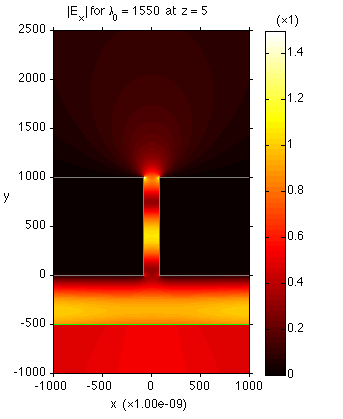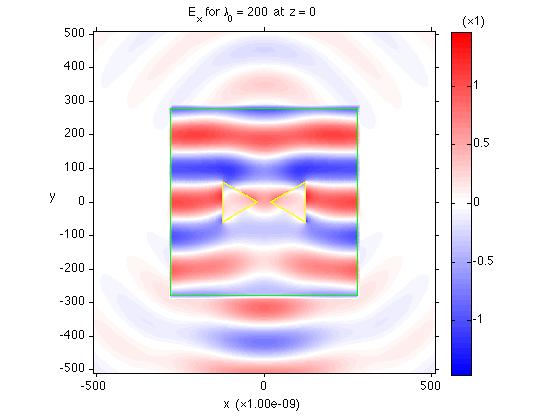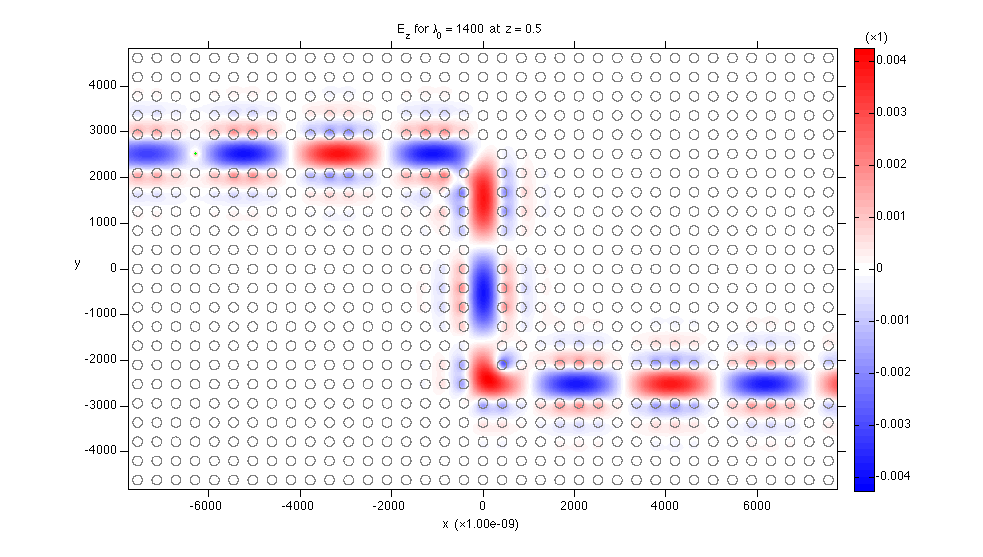# MaxwellFDFD

MaxwellFDFD is a MATLAB-based package that solves the frequency-domain Maxwell's equations

$\nabla \times \mathbf{E}(\mathbf{r}) = -i \, \omega \, \mu(\mathbf{r},\mathbf{\omega}) \, \mathbf{H}(\mathbf{r}) - \mathbf{M}(\mathbf{r}),\\ \nabla \times \mathbf{H}(\mathbf{r}) = i \, \omega \, \varepsilon(\mathbf{r},\mathbf{\omega}) \, \mathbf{E}(\mathbf{r}) + \mathbf{J}(\mathbf{r}),$

where

• $$\mathbf{r}$$ is the position variable;

• $$\mathbf{E}(\mathbf{r})$$ and $$\mathbf{H}(\mathbf{r})$$ are the solution electric and magnetic fields of the EM waves;

• $$\mathbf{J}(\mathbf{r})$$ and $$\mathbf{M}(\mathbf{r})$$ are the electric and magnetic current source densities emanating the EM waves;

• $$\omega$$ is the oscillation frequency of the current sources; and

• $$\varepsilon(\mathbf{r},\omega)$$ and $$\mu(\mathbf{r},\omega)$$ are the electric permittivity and magnetic permeability of the object at $$\mathbf{r}$$ for $$\omega$$.

MaxwellFDFD solves the above Maxwell's equations by the finite-difference frequency-domain (FDFD) method, and hence the name MaxwellFDFD.

## Major Features

• Built-in frequency-dependent dielectric constants for commonly used nanophotonic materials (e.g., Ag, Au, Si, SiO₂) taken from the widely used references (e.g., Johnson and Christy, Palik, CRC Handbook)

• Dynamic nonuniform grid generation aligned with object boundaries

• Waveguide mode solver and waveguide-mode-generating current source

• 2D and 3D visualization of objects, sources, and solution fields

• Periodic array of objects

• Electric ($$\mathbf{J}$$) and magnetic ($$\mathbf{M}$$) current sources

• PEC, PMC, periodic, and Bloch boundary conditions

• Stretched-coordinate perfectly matched layer (SC-PML) and uniaxial PML (UPML)

• Total-field/scattered-field (TF/SF) method

• Power flux calculation

## System Requirements

• MATLAB 2014a or later (no Toolbox needed)

Press the “Download ZIP” button in the right panel of MaxwellFDFD's GitHub page.

## Documentation

The online documentation is available here. (The entire MATLAB-style documentation is included in the doc/ directory of the downloaded MaxwellFDFD package.)

## Examples

• Plane wave incident on a slit in a silver slab• TF/SF simulation of a plane wave incident on a gold bow-tie antenna• Point source in a photonic crystal waveguide double bend## Basic Usage

Using MaxwellFDFD is very simple. There is an all-in-one function, maxwell_run(), and you just need to fill in its input parameters. For example, below is the code for the first example shown above.

[E, H] = maxwell_run(...
'OSC', 1e-9, 1550, ...
'DOM', {'vacuum', 'none', 1.0}, [-1100 1100; -1100 2600; 0 10], 10, BC.p, [100 100 0], ...
'OBJ', {'CRC/Ag', 'k'}, ...
Box([-1100 -80; 0 1000; 0 10]), ...
Box([80 1100; 0 1000; 0 10]), ...
'SRCJ', PlaneSrc(Axis.y, -500, Axis.x));


The meaning of the input parameter groups used in the code is as follows:

• 'OSC' (oscilation): the length unit (1 nm) and free-space wavelength (1550 nm)

• 'DOM' (domain): the material and size of the simulation domain

• 'OBJ' (object): the materials and shapes of the objects in the simulation domain

• 'SRCJ' (source): the electric current source in the simulation domain

The output parameters E and H are the solution E- and H-fields of the simulation.

## How to Cite

You are welcome to cite the following paper about the techniques used in MaxwellFDFD:

• W. Shin and S. Fan, “Choice of the perfectly matched layer boundary condition for frequency-domain Maxwell's equations solvers,” Journal of Computational Physics, vol. 231, pp. 3406–3431 (2012) [pdf]

You can also cite the URL of MaxwellFDFD's GitHub page as follows:

• W. Shin, MaxwellFDFD Webpage, 2015. https://github.com/wsshin/maxwellfdfd

If you use LaTeX, here is the BibTeX record for the GitHub page:

@webpage{maxwellfdfd-webpage,
Author = {Wonseok Shin},
Note = {{https://github.com/wsshin/maxwellfdfd}},
Title = {{MaxwellFDFD} {W}ebpage},
Year = {2015}
}